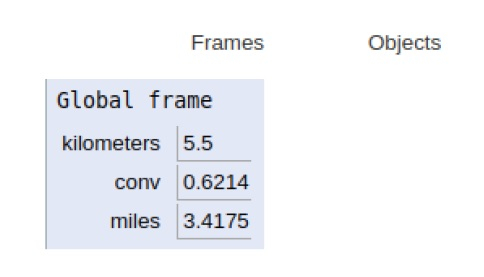# Python Program to convert Kilometers to Miles

PythonServer Side ProgrammingProgramming

Problem statement − We are given distance in kilometers and we need to convert it into miles

As we know that 1 kilometer equals 0.62137 miles.

## Formula Used

Miles = kilometer * 0.62137

Now let’s observe the concept in the implementation below−

## Example

Live Demo

kilometers = 5.5
# conversion factor as 1 km = 0.621371 miles
conv = 0.621371
# calculation
miles = kilometers * conv
print(kilometers,"kilometers is equal to ",miles,"miles")

## Output

5.5 kilometers is equal to 3.4175405 milesAll the variables are declared in the local scope and their references are seen in the figure above.